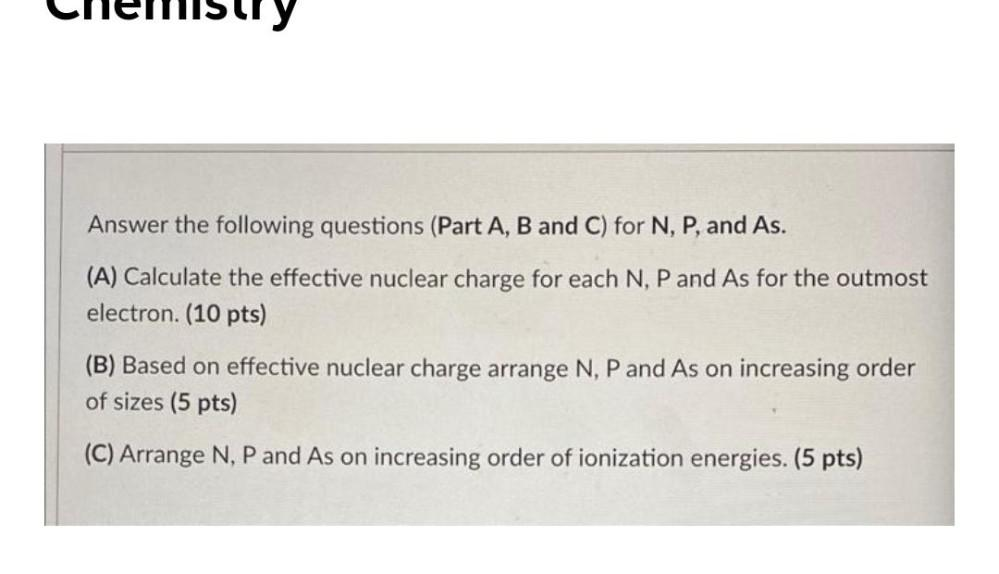Question:

# Answer the following questions (Part A, B and C) for N, P, and As. (A) Calculate the effective nuclear charge for each N, P andAnswer the following questions (Part A, B and C) for N, P, and As. (A) Calculate the effective nuclear charge for each N, P and As for the outmost electron. (10 pts) (B) Based on effective nuclear charge arrange N, P and As on increasing order of sizes (5 pts) (C) Arrange N, P and As on increasing order of ionization energies. (5 pts)